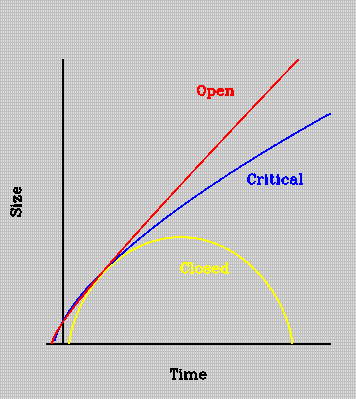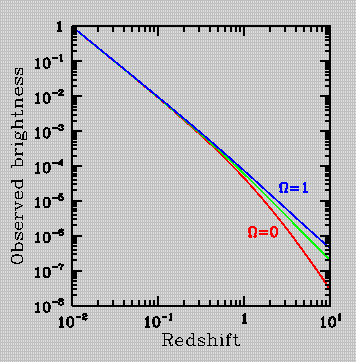# The Hubble Expansion

During the 1920's and 30's, Edwin Hubble discovered that the Universe is expanding, with galaxies moving away from each other at a velocity given by an expression known as Hubble's Law: v = H*r. Here v represent's the galaxy's recessional velocity, r is its distance away from Earth, and H is a constant of proportionality called Hubble's constant.

The exact value of the Hubble constant is somewhat uncertain, but is generally believed to be between 50 and 100 kilometers per second for every megaparsec in distance, km/sec/Mpc. (A megaparsec is given by 1 Mpc = 3 x 10^6 light-years). This means that a galaxy 1 megaparsec away will be moving away from us at a speed of between 50 and 100 km/sec, while another galaxy 100 megaparsecs away will be receding at 100 times this speed. So essentially, the Hubble constant sets the rate at which the Universe is expanding.

Additionally, the present age of the Universe can be assessed vis-a-vis the Hubble constant: the inverse of the Hubble constant has units of time. By substituting in kilometers for Mpc in the Hubble constant, we find that upon inverting H we get a quantity with units of seconds (kilometers canceling out in the denominator and numerator). For a Hubble constant of 100 kilometers per second per Mpc, we get 3 x 10^7 seconds, or about 10 billion years. For H=50 kilometers per second per Mpc, the time scale is 20 billion years.The size of the universe as a function of time. In the case of a closed universe there is enough matter that gravity halts the expansion and the universe recollapses (and perhaps bounces to repeat the process). In an open universe there is not enough matter to halt the expansion and the universe expands forever, becoming more and more dilute as time passes. The border between recollapsing and expanding forever is called a critical universe.

The standard picture of cosmology, based on Einstein's general theory of relativity explains how to picture this expanding universe. As an example consider a loaf of bread, with raisins sprinkled evenly throughout it. As the bread expands during cooking all the raisins are moved further and further apart from each other. Seen from any raisin all the other raisins in the bread appear to be receding with some velocity.

This model also explains the linearity of the Hubble law, by which we mean the fact that the recession velocity is proportional to distance. If all the lengths in the universe double in 10 million years then something that was initially 1 megaparsec away from us will end up a further megaparsec away. Something that was 2 megaparsecs away from us will end up a further 2 megaparsecs away. In terms of the speed at which the objects appear to be receding from us, the object twice as distant has receded twice as fast!

On very large scales Einstein's theory predicts departures from a stricly linear Hubble law. The amount of departure, and the type, depends on the value of the total mass of the universe. In this way a plot of recession velocity (or redshift) vs. distance (a Hubble plot), which is a straight line at small distances, can tell us about the amount of matter in the universe and provide crucial information about dark matter.The brightness of an object seen at various distances in the universe. The object has been arbitrarily chosen to have brightness 1 at redshift z=0.01 (about 60Mpc away). For small distances the brightness falls with the square of the distance, since we see less and less of the total light put out by the object. At larger distances the curvature of the universe, which depends on how much matter it contains, starts to play a role. In a high Omega universe objects at fixed redshift appear brighter than in a lower density universe, because they are closer. This effect becomes more and more important at higher redshift. Note that Astronomers often plot such a diagram in magnitudes vs redshift.

A translation of this page is available in French and again in French.
Emad Iskander, Douglas Scott & Martin White